Request your copy of SimphoSOFT® now

Webinars
Product brochureApplication notesThree-photon absorption (3PA) is a nonlinear absorption process whereby three photons are absorbed simultaneously by an atom, ion or molecule and an electron is promoted from a lower energy level to a higher energy level. For example, three-photon absorption can excite an electron from the ground state to an excited state. The total energy of the transition is equal to the sum of the three photon energies.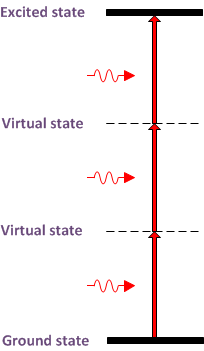The equation describing the intensity attenuation of a light beam passing through a material undergoing single-photon, two-photon and three-photon absorption is given by

 ∂I  / ∂z = − α I − β I2 − γ I3 (prop equation)

where α, β and γ are constants. The constant γ for three-photon absorption is related to the fifth-order susceptibility. The absorbed power in a thickness element dz for single-photon absorption is proportional to light intensity, whereas the absorbed power for two-photon absorption is proportional to the intensity squared and the absorbed power for three-photon absorption is proportional to the intensity cubed.

Three-photon absorption constant γ is usually found for a certain (known) concentration of photo-activated material (species). The main assumption is that during interaction of laser beam with the material the concentration of photo-activated species (molecules, ions, QDs etc) remains constant most of the time (e.g., it reaches equilibrium in a fraction of the beam duration). However, for ultra-fast processes this assumption is no longer valid and a more detailed model is usually used for numerical calculations. The central parameter of the new model is a three-photon absorption molecular cross-section σ3PA , while the concentration (or, population density) of the species being in ground state N0 is is “detached” from the absorption constant as follows:

 ∂I(z,t)  / ∂z = − σ3PA N0(z,t) I3(z,t) (detailed PROP)

For simplicity, we left many other important terms out of this example equation – which may include single-photon, two-photon, or, generally speaking, multi-photon absorption, linear absorption, stimulated emission etc. Each multi-photon absorption term will have a factor of population density of the energy state from which the absorption occurs. The function of concentration N0(z,t) follows the following corresponding rate equation:

 ∂N0  / ∂t = − σ3PA N0(z,t) I3(z,t) /3 h ω0 + k10 (N-N0) (RATE equation)

where N is the initial concentration of species and k10 attributes to the rate of relaxation of species from the excited state.SimphoSOFT® supports modeling two-photon (as well as single- and three-photon) absorption. Its mathematical model includes propagation equation, written in the terms of electromagnetic field, coupled with time-resolved radially-dependent rate equations.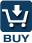SimphoSOFT® can be purchased as a single program and can be also configured with Energy Transfer add-on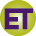, Multi-Beam add-on, Optimization add-on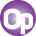, Z-scan add-on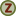, and MPA Info+ add-onfor an additional charge. Please, contact our sales staff for more information e-mail phone: +1 (973) 621-2340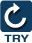You can request a remote evaluation of our software by filling out online form -- no questions asked to get it, no need to install it on your computer.